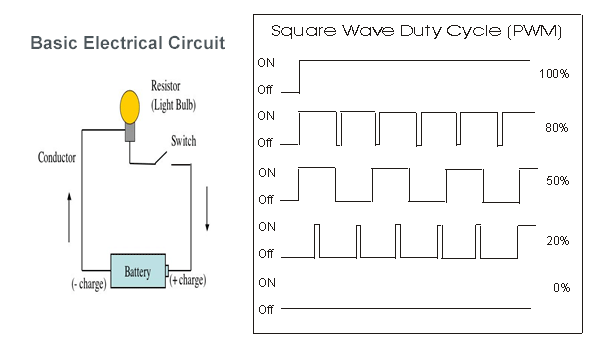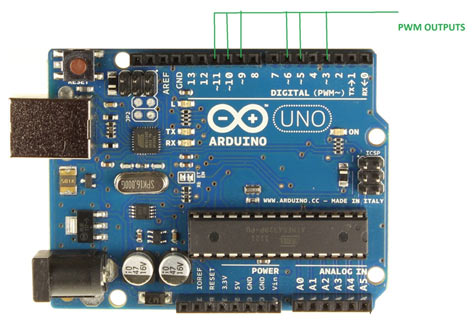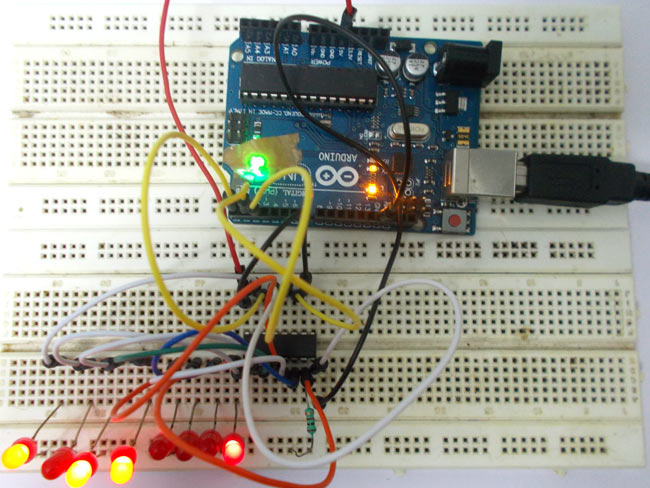# Arduino Based LED Dimmer using PWM

This LED DIMMER is an Arduino Uno based PWM (Pulse Width Modulation) circuit developed to get variable voltage over constant voltage. The method of PWM is explained below.Before we get start building a 1 Watt LED Dimmer circuit, first consider a simple circuit as shown in figure below.Now if the switch in the figure is closed continuously over a period of time then the bulb will continuously ON during that time. If the switch is closed for 8ms and opened for 2ms over a cycle of 10ms, then the bulb will be ON only in the 8ms time. Now the average terminal over across the over a period of 10ms = Turn ON time/ (Turn ON time + Turn OFF time), this is called duty cycle and is of 80% (8/ (8+2)), so the average output voltage will be 80% of the battery voltage.

In the second case, the switch is closed for 5ms and opened for 5ms over a period of 10ms, so the average terminal voltage at the output will be 50% of the battery voltage. Say if the battery voltage is 5V and the duty cycle is 50% and so the average terminal voltage will be 2.5V.

In the third case the duty cycle is 20% and the average terminal voltage is 20% of the battery voltage.

Now how this technique is used in this LED Dimmer? It is explained in the subsequent section of this tutorial.As shown in figure, an Arduino UNO has 6PWM channels, so we can get PWM (variable voltage) at any of these six pins. In this chapter we are going to use PIN3 as PWM output.

READ  Arduino color picker reveals real world color RGB values

### Required Components

Hardware: ARDUINO UNO, power supply (5v), 100uF capacitor ,LED, buttons (two pieces), 10KΩ resistor (two pieces).

Software: arduino IDE

Read More:  Arduino Based LED Dimmer using PWM

#### This Post / Project can also be found using search terms:

• arduino using pwm to dim led
• using arduino as dimmer switch
• arduino dimmer led pwm
• arduino led dimmer